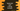# 3 different ways to split a string in typescript## How to split a string in typescript:

Splitting a string is one of the most commonly used operation. TypeScript provides one inbuilt method split that can be used to split a string. In this post, we will learn how to use the split method with different examples.

### Definition of split:

The split method is defined as below:

``str.split(separator: string | regExp,limit?: number | undefined)``

Here, separator is the separator parameter or a regular expression used for the splitting and limit is the limit we want for that splitting. limit is an integer value defines the limits of the number of splits.

This method returns an array containing the strings.

### Example 1: Split a string without using separator and limit:

Let’s take a look at the below example program:

``````const givenStr = "The quick brown fox jumps over the lazy dog"

const splittedArray = givenStr.split(" ")

console.log(splittedArray)``````

It will print:

``["The", "quick", "brown", "fox", "jumps", "over", "the", "lazy", "dog"]``

### Example 2: Split a string with a regular expression:

``````const givenStr = "one2two3three4four5five6six"
const pattern = new RegExp('[0-9]')

const splittedArray = givenStr.split(pattern)

console.log(splittedArray)``````

This example uses one regular expression that matches all numbers from 0 to 9. The splitting is done in numbers for this string.

If you run it, it will print the below output:

``["one", "two", "three", "four", "five", "six"]``

### Example 3: Split a string with limit:

Let’s try with the second parameter now: limit. This is a number to define the number of splits that we want. For example, if I pass 3 as this variable in the above program:

``````const givenStr = "one2two3three4four5five6six"
const pattern = new RegExp('[0-9]')

const splittedArray = givenStr.split(pattern,3)

console.log(splittedArray)``````

It will return only the first three words:

``["one", "two", "three"]``

### JavaScript conversion:

This method is actually the same one that we use in JavaScript. If I compile the above program to JavaScript, it will be :

``````"use strict";
const givenStr = "one2two3three4four5five6six";
const pattern = new RegExp('[0-9]');
const splittedArray = givenStr.split(pattern, 3);
console.log(splittedArray);``````